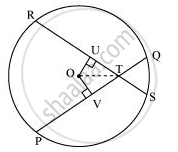# If Two Equal Chords of a Circle Intersect Within the Circle, Prove that the Segments of One Chord Are Equal to Corresponding Segments of the Other Chord. - Mathematics

If two equal chords of a circle intersect within the circle, prove that the segments of one chord are equal to corresponding segments of the other chord.

#### Solution

Let PQ and RS be two equal chords of a given circle and they are intersecting each other at point T.Draw perpendiculars OV and OU on these chords.

In ΔOVT and ΔOUT,

OV = OU (Equal chords of a circle are equidistant from the centre)

∠OVT = ∠OUT (Each 90°)

OT = OT (Common)

∴ ΔOVT ≅ ΔOUT (RHS congruence rule)

∴ VT = UT (By CPCT) ... (1)

It is given that,

PQ = RS ... (2)

⇒ 1/2PQ = 1/2RS

⇒ PV = RU ... (3)

On adding equations (1) and (3), we obtain

PV + VT = RU + UT

⇒ PT = RT ... (4)

On subtracting equation (4) from equation (2), we obtain

PQ − PT = RS − RT

⇒ QT = ST ... (5)

Equations (4) and (5) indicate that the corresponding segments of chords PQ and RS are congruent to each other.

Concept: Equal Chords and Their Distances from the Centre
Is there an error in this question or solution?

#### APPEARS IN

NCERT Class 9 Maths
Chapter 10 Circles
Exercise 10.4 | Q 2 | Page 179